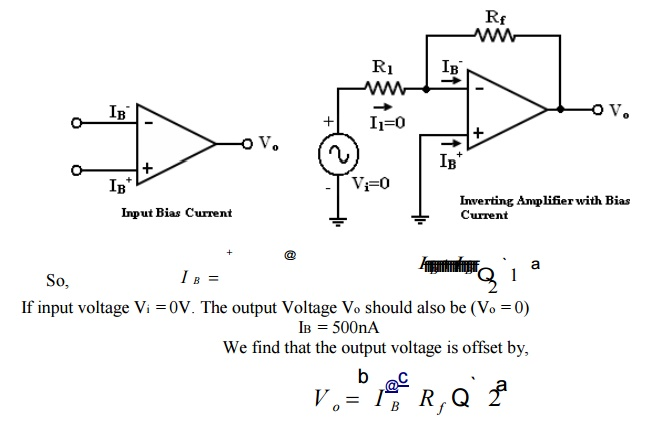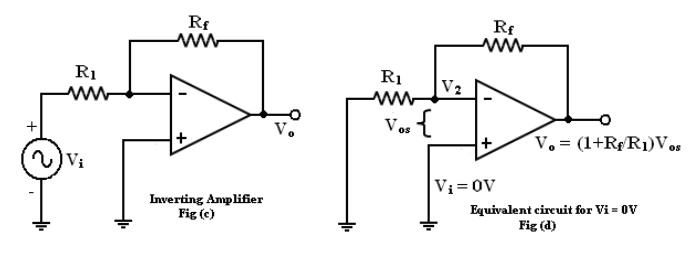Home | | Linear Integrated Circuits | DC Characteristics of op-amp

# DC Characteristics of op-amp

Current is taken from the source into the op-amp inputs respond differently to current and voltage due to mismatch in transistor. DC output voltages are, 1. Input bias current 2. Input offset current 3. Input offset voltage 4. Thermal drift

DC Characteristics of op-amp:

Current is taken from the source into the op-amp inputs respond differently to current and voltage due to mismatch in transistor.

DC output voltages are,

1.        Input bias current

2.        Input offset current

3.        Input offset voltage

4.        Thermal drift

1. Input bias current:

The op-amp‘s input is differential amplifier, which may be made of BJT or FET.

·            In an ideal op-amp, we assumed that no current is drawn from the input terminals.

·            The base currents entering into the inverting and non-inverting terminals (IB-& IB+ respectively).

·            Even though both the transistors are identical, IB- and IB+ are not exactly equal due to internal imbalance between the two inputs.

·            Manufacturers specify the input bias current IBOp-amp with a 1M feedback resistor

V0 = 5000nA X 1M = 500mV

The output is driven to 500mV with zero input, because of the bias currents.

In application where the signal levels are measured in mV, this is totally unacceptable. This can be compensated. Where a compensation resistor Rcomp has been added between the non-inverting input terminal and ground as shown in the figure below.Current IB+ flowing through the compensating resistor Rcomp, then by KVL we

-V1+0+V2-Vo = 0 (or)

Vo = V2 – V1 ——>(3)

By selecting proper value of Rcomp, V2 can be cancelled with V1 and the Vo = 0. The value of Rcomp is derived a

V1  =I=+Rcomp                  (or)

IB+ = V1/Rcomp  ——>(4)

The node =a‘ is at voltage (-V1). Because the voltage at the non-inverting input terminal is (-V1). So with Vi = 0 we get,

I1 = V1/R1 ——>(5)

I2 = V2/Rf ——>(6)

For compensation, Vo should equal to zero (Vo = 0, Vi = 0). i.e. from equation (3) V2 = V1. So that,

I2 = V1/Rf ——>(7)

KCL at node =a‘ gives,

IB-= I2 + I1

IB- = RfR1

Assume IB- = IB+ and using equation (4) & (8) we get

Rcomp = R1 + Rf

Rcomp = R1 || Rf               --->(9)

i.e. to compensate for bias current, the compensating resistor, Rcomp combination of resistor R1 and Rf.

2. Input offset current:

Bias current compensation will work if both bias currents IB+ and IB- are equal.

Since the input transistor cannot be made identical. There will always be some small difference between IB+ and IB-. This difference is called the offset current

|Ios| = IB+ -IB-        -à(10)

Offset current Ios for BJT op-amp is 200nA and for FET op-amp is 10pA. Even with bias current compensation, offset current will produce an output voltage when Vi = 0.Equation (16) the offset current can be minimized by keeping feedback resistance small.

·            Unfortunately to obtain high input impedance, R1  must be kept large.

·            R1  large, the feedback resistor Rf must also be high. So as to obtain reasonable gain.

The T-feedback network is a good solution. This will allow large feedback resistance, while keeping the resistance to ground low (in dotted line).

·           The T-network provides a feedback signal as if the network were a single feedback resistor.

By T to Π conversion,

To design T- network first pick Rt<<Rf/23. Input offset voltage:

Inspite of the use of the above compensating techniques, it is found that the output voltage may still not be zero with zero input voltage [Vo ≠ 0 with Vi = 0]. This is due to unavoidable imbalances inside the op-amp and one may have to apply a small voltage at the input terminal to make output (Vo) = 0.

This voltage is called input offset voltage Vos. This is the voltage required to be applied at the input for making output voltage to zero (Vo = 0).Let us determine the Vos on the output of inverting and non-inverting amplifier. If Vi = 0 (Fig (b) and (c)) become the same as in figure (d).

Total output offset voltage:

The total output offset voltage VOT could be either more or less than the offset voltage produced at the output due to input bias current (IB) or input offset voltage alone(Vos).

This is because IB and Vos could be either positive or negative with respect to ground. Therefore the maximum offset voltage at the output of an inverting and non-inverting amplifier (figure b, c) without any compensation technique used is given by many op-amp provide offset compensation pins to nullify the offset voltage.

·            10K potentiometer is placed across offset null pins 1&5. The wipes connected to the negative supply at pin 4.

·           The position of the wipes is adjusted to nullify the offset voltageWhen  the  given  (below)  op-amps  does  not  have  these  offset  null  pins,  external  balancing techniques are used

Balancing circuit:

Inverting amplifier:Non-inverting amplifier:4. Thermal drift:

·            Bias current, offset current, and offset voltage change with temperature.

·            A circuit carefully nulled at 25ºC may not remain. So when the temperature rises to 35ºC. This is called drift.

·            Offset current drift is expressed in nA/ºC.

·            These indicate the change in offset for each degree Celsius change in temperature.

Study Material, Lecturing Notes, Assignment, Reference, Wiki description explanation, brief detail
Linear Integrated Ciruits : Characteristics of op-amp : DC Characteristics of op-amp |